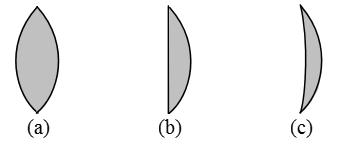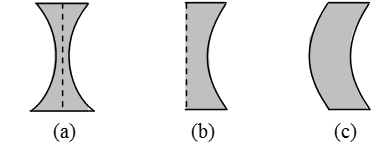# Physics

### Chapter : 4. Light Refraction

#### Spherical Lens

Spherical Lens
Definition : A piece of a transparent medium bounded by at least one spherical surface, is called a spherical lens.
Types : There are two types of spherical lenses:
(i) Convex or Converging Lenses : These are thick in the middle and thin at the edges.Fig. Three types of convex lenses
(a) Double Convex Lens : It has both the surfaces convex.
(b) Plano–Convex Lens : It has one surface plane and the other surface convex.
(c) Concavo–Convex Lens : It has one surface concave and the other surface convex.
(ii) Concave or Diverging Lenses : These are thin in the middle and thick at the edges.
There are three types of concave lenses :Fig. Three types of concave lenses
(a) Double Concave Lens : It has both the surfaces concave. (Fig.)
(b) Plano–Concave Lens : It has one surface plane and the other surface concave. (fig.)
(c) Convexo–Concave Lens : It has one surface convex and the other surface concave. (fig.)